| Technical Review: ABCOM Team | Level: Advanced | Banner Image Source : Internet |

# Background

In the previous part (Part - 1) of this series, you learned how to select the nodes and its desired attributes for analysis of a humongous network, be it a social one like Facebook or your company’s own network. In part 1, I showed you how to generate different network visualization plots that give you insights on the locality and density of the network. In part 2 of this three-part tutorial, I will show you how to analyze the network to do predictions such as the most likely future associations at any node. We call this link prediction; we will use the Node2Vec package for this analysis and prediction. Here outlines what I cover in this tutorial.

1. Background Information :
• Where is it Used?
2. Developing Application :
• Processing data
• Building model
• Choosing the best model
• Inference

I will first define few terms for you to understand what is a link prediction.

# Future Associations

Because of the dynamics of a social network, we stumble upon questions like; How are the links between nodes formed? How do these links change over time? Which nodes will form future connections? Taking into consideration all these factors, we need to predict the likelihood of a future association.

Link prediction algorithms are based on how similar two different nodes are, what features they have in common, how are they connected to the rest of the network, how many other nodes are connected to a single node, etc. Answers to such questions help us in predicting the future connections of a node and also find the missing links in an incomplete graph.

Link prediction finds its usage in many areas like recommendation systems, social networks, information retrieval, and so on. In social networks we use it as a ‘friend finder’, i.e. ‘People you may know’ section on Facebook. In e-commerce applications, the link predictions are used to build recommendation systems like the ‘People who bought this also bought’ feature on Amazon. We can use a bioinformatics network for finding interactions between proteins. In extreme situations, such analysis may also help to find hidden groups of terrorists or criminals. The link prediction using AI techniques helps us in finding connections faster and in advance, which otherwise might have taken a long time to form serendipitously.

For analysis of such networks, we use similarity measures between the nodes. There are several concepts and mathematical indices used for such measurements. Some of these are:

• Common Neighbors
• Jaccard Coefficient
• Resource Allocation Index
• Preferential Attachment
• Community Common Neighbors
• Community Resource Allocation Index

We can use the above indices as features for our model. I will cover these in more depth and practical use in part three of this series. Let us now focus on link prediction using node2vec in this part.

# Creating Project

Create a new Colab project and rename it SNA-II. Import the required libraries into your project:

``````import random
from tqdm import tqdm
import networkx as nx
from zipfile import ZipFile
import pandas as pd
import numpy as np
import matplotlib.pyplot as plt
%matplotlib inline
from warnings import simplefilter
from sklearn.exceptions import ConvergenceWarning
simplefilter("ignore", category=ConvergenceWarning)
``````

You need the dataset used in the first part of this series. At the end of the first part (part 1), you had saved two data files, which I said would be required for part 2. We download these files in the current project using the following program code:

``````!wget https://github.com/abcom-mltutorials/Facebook-Social-Network-Analysis/archive/master.zip -P "/content"
ZipFile("/content/master.zip").extractall("/content/")

# graph
# fb dataframe
``````

Just to recapitulate what it contains, print the network info:

``````print(nx.info(G))
``````

This is the output:

``````Name: Facebook SNA Graph
Type: Graph
Number of nodes: 1295
Number of edges: 18321
Average degree:  28.2950
``````

The output shows the number of nodes, edges and the average connectivity in our selected dataset. Print the `fb` dataset read from fb.csv file:

``````fb
``````

This gives the following output: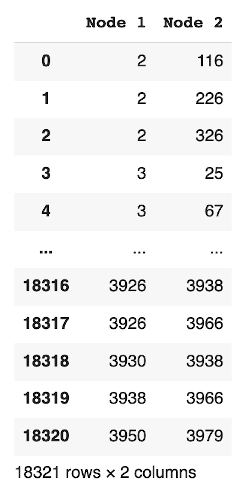It shows that we have totally 18000 odd records showing the connection between two nodes in the network.

We will now process the data in this network for our desired analysis of future associations.

# Creating new Graph

As you have now loaded the network graph from the previous part, we will do some processing on it for our prediction analysis. At the end of this section, you will have a new graph which will be used as a dataset for neural network modeling.

First, we will create an adjacency matrix for the network.

This is how Wikipedia defines an adjacency matrix.

‘In graph theory and computer science, an adjacency matrix is a square matrix used to represent a finite graph. The elements of the matrix indicate whether pairs of vertices are adjacent or not in the graph. In the special case of a finite simple graph, the adjacency matrix is a (0,1) - matrix with zeros on its diagonal.’ - Wikipedia, Adjacency Matrix

Simply put, an adjacency matrix is a matrix which contains both rows and columns as the nodes in the graph. For certain nodes i and j, if there is an edge between them, then the adjacency matrix A[i,j] will be equal to 1, and if there dose not exist an edge between them, then A[i,j] will be 0.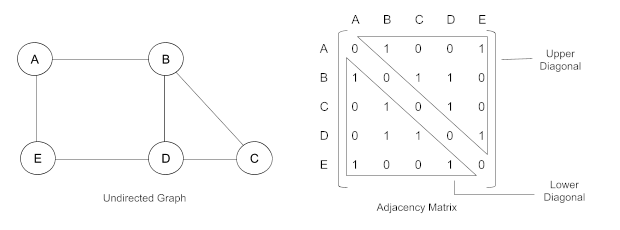For example, in the above diagram, we can see that there is an edge between A and B, while there doesn’t exist an edge between A and C, hence their values in the graph are 1, 0 respectively.

We will create such a matrix for our graph. As you can see from the above example, the upper diagonal of the matrix (elements above the diagonal of the matrix) is equal to the lower diagonal (elements below the diagonal of the matrix). This is always the case for any adjacency matrix. Thus, we need to generate only one diagonal in our computation.

Having seen what is an adjacency matrix, we generate the one for our network:

``````# get a list of nodes in our graph
l = list(G.nodes())

adj_G = nx.to_numpy_matrix(G, nodelist = l)

``````

The output is as follows: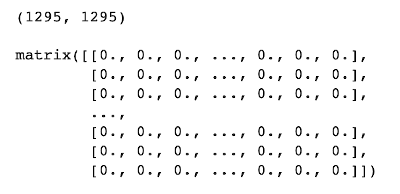We see that the matrix is a square matrix of size 1295x1295. All the displayed elements in the above screenshot have value 0, showing that the nodes at corresponding indices are not connected. Had the value been 1, it would indicate that those two corresponding nodes are connected.

Next, we will create a Python list of all such non-connected node pairs, having value 0 in the adjacency matrix.

## Finding Non-Connected Nodes

To get a list of non-existing edges (nodes which are not connected), we need to find out the elements in our adjacency matrix that have value 0. We call this list `non_existing_edges`. We need to traverse only one half of the matrix - upper or lower, as both have identical element values. Also, we ignore the diagonal elements - a diagonal element shows a node having an edge with itself and thus always have a value of 0 for each such element.

``````# get all node pairs which don't have an edge
non_existing_edges = []

offset = 0
if i != j:
non_existing_edges.extend([(l[i],l[j])])

offset = offset + 1
``````

The output is as follows:

``````[(2, 3), (2, 7), (2, 10), (2, 13), (2, 16)]
``````

The output shows that nodes 2 and 3 do not have an edge between them. Likewise, nodes 2 and 16 do not have an edge between them. Check the number of such node pairs by calling the `len` function:

``````len(non_existing_edges)
``````

It shows that you have 819,544 such pairs, which is a fairly large number for non-connected nodes.

## Getting a Subset of Non-Connected Nodes

We have lots of non existing edges in our graph, to maintain a good ratio between existing and non-existing edges, we will select only a certain number of non-existing edges for our analysis. We need to balance the dataset for better learning of our neural network model. Note that in machine learning to train the model, you need to have a balance between positive and negative data points.

We will randomly select 4000 non-existing edges from the vast collection of 800K+ edges.

``````nodes_4000 = sorted(random.sample(non_existing_edges, k=40000))
``````

Next, we search for connected nodes in this list.

## Finding Connected Nodes

If we can reach a node through other nodes, then we consider that these two nodes are connected by a well-defined path. The nodes for which a path does not exist carry a little likelihood of a connection in near future. We can safely eliminate such nodes in our further analysis. To sort out nodes with a reachable connection path, we use the following loop:

``````non_existing_edges = [(i,i) for i in tqdm(nodes_4000) if nx.has_path(G, i, i)]
``````

Try printing a few records:

``````non_existing_edges[:5]
``````

The output is as follows:

``````[(2, 7), (2, 25), (2, 55), (2, 119), (2, 146)]
``````

The output shows that the nodes 2 and 7 have a path between them. Similarly, there is a path between nodes 119 and 2. Note that these nodes do not have a direct connection to each other; however, they are connected through a few interconnected nodes. It is as good as saying, “friend’s friend’s friend may become your friend.”

## Creating Dataframe of Non_Existing_Edges

Now, as a part of further data processing, let us create a new dataframe of the `non_existing_edges`, and call it `df1`. A data frame would help us in adding and interpreting those edges easily. We make a column in the data frame called Connection and set all its values to 0, denoting that there doesn’t exist an edge between all these node pairs. These are our negative samples.

``````df1 = pd.DataFrame(data = non_existing_edges, columns =['Node 1', 'Node 2'])

# create a column 'Connection' with default 0 (no-connection)
df1['Connection'] = 0

``````

This is the output: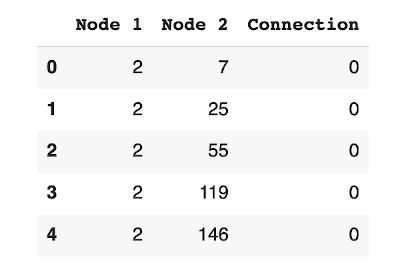You can see from the output that the nodes 2 and 7 do not have an edge between them, hence the value 0 in the corresponding connection column. Same goes for the rest of the nodes.

## Getting Removable Edges

Next, we create a list of the indices of the node pairs in the `fb` data frame, which when removed will not change the graph’s structure. We call these removable node pairs/edges. Such edges are not required in our analysis and should be removed from the graph for better performance.

To get such removable edges from the graph, you will scan through all node pairs, remove an edge, check its effect on the number of connected components and the nodes. If these figures do not change in the original graph, we mark such an edge for removal. After collecting all such edges, we will eventually remove those from our graph. I do this in the following code snippet:

``````# Create a list of all indices of the node pairs in the fb dataframe,
# which when removed won’t change the structure of our graph

# create a copy
fb_temp = fb.copy()

# for storing removable edges
removable_edges_indices = []

# number of connected components and nodes of G
ncc = nx.number_connected_components(G)
number_of_nodes = len(G.nodes)

# for each node pair we will be removing a node pair and creating a new graph,
# and check if the number of connected components and the number of nodes
# are the same as the original graph
for i in tqdm(fb.index.values):

# remove a node pair and build a new graph
G1 = nx.from_pandas_edgelist(fb_temp.drop(index= i), "Node 1", "Node 2",
create_using=nx.Graph())

# If the number of connected components remain same as the original
# graph we won't remove the edge
if (nx.number_connected_components(G1) == ncc) and (len(G1.nodes) == number_of_nodes):
removable_edges_indices.append(i)

# drop the edge, so that for the next iteration the next G1
# is created without this edge
fb_temp = fb_temp.drop(index = i)
``````

In the preceding code, first we make a copy of the `fb` data frame called `fb_temp`. We declare an array called `removable_edges_indices` for storing the removable edges. We record the number of connected components and nodes in the existing graph in two variables - `ncc` and `number_of_nodes`. We iterate over the entire range of nodes using `tqdm`.

``````for i in tqdm(fb.index.values):
``````

We remove a node by calling the `from_pandas_edgelist` method creating a new graph on each iteration:

``````   G1 = nx.from_pandas_edgelist(fb_temp.drop(index= i), "Node 1", "Node 2",
create_using=nx.Graph())
``````

The Node 1 and Node 2 parameters name the source and destination graphs. We check if the number of components and nodes is affected; if not, we mark the corresponding edge for removal.

``````   if (nx.number_connected_components(G1) == ncc) and (len(G1.nodes) == number_of_nodes):
removable_edges_indices.append(i)
``````

We also drop this edge so that in the next iteration, this edge is not reconsidered for removal.

``````       fb_temp = fb_temp.drop(index = i)
``````

After the loop ends, you can check the collected list by printing its few items.

``````removable_edges_indices[:5]
``````

The output is as follows

``````[0, 1, 3, 4, 5]
``````

The output shows that the node pairs having index (in the `fb` data frame) 0, 1, 3, and so on can be removed without changing the graph’s structure.

## Creating Dataframe of Removable Edges

Next, we will create a data frame `df2` which contains all the removable edges. As earlier with `df1`, we create a Connection column and set its value to 1, which means the node pair has an edge between them.

``````# get node pairs in fb dataframe with indices in removable_edges_indices
df2 = fb.loc[removable_edges_indices]

# create a column 'Connection' and assign default value of 1 (connected nodes)
df2['Connection'] = 1

``````

The output is as follows: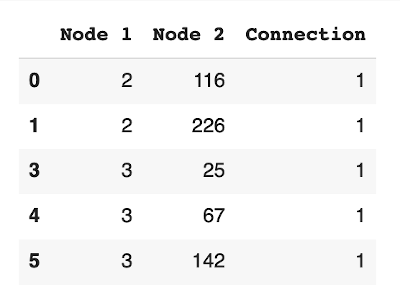You can see from the output that the nodes 2 and 116 have an edge between them, hence the corresponding value in the connection column is 1. Similarly nodes 3 and 142 are also connected by an edge.

## Creating Subgraph

We will now create a subgraph of our original graph `fb` by dropping the combined data frames `df1` and `df2`. We combine the two data frames `df1` and `df2`:

``````df1 = df1.append(df2[['Node 1', 'Node 2', 'Connection']],
ignore_index=True)
``````

The updated data frame contains all connected nodes having an edge denoted by connection value of either 0 or 1. You may print `df1` to examine its contents.

``````df1.head()
``````

This is the output: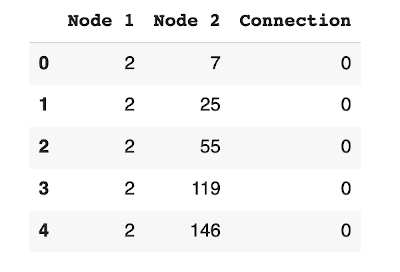We want to create a graph which may have been existing at a previous point of time. How do we do this? We have already created a list of removable edges which when removed won’t change the structure of our graph. So, we will remove these ‘removable edges’ from our original graph ‘G’ and thus create a new graph, let’s say ‘G_new’. Now ‘G’ becomes our present graph and ‘G_new’ becomes a graph that may have been existing in the past. Hence ‘G_new’ has some node pairs which don’t have an edge between them, but for that same node pair there may be an edge in ‘G’. Now, let us time travel a little, let us say that ‘G_new’ is the graph that is present now. Then that makes ‘G’ the graph which will be present in the future. Hence, again assuming that ‘G_new’ is our present graph, we now know which nodes will form a connection/edge in the future, i.e. the graph ‘G’. And the connection column in `df1` contains this information only, where 0 means the node pair that does not have an edge, while 1 means the node pair has an edge, in the future, i.e. in the graph ‘G’.

Now all we have to do is to get the features of the nodes from graph ‘G_new’, and predict the possibility of the nodes forming an edge in the future, i.e. the graph ‘G’, using the connection column of `df1` as our target feature.

In the next cell, we have created a new data frame `df3` which contains the remaining edges, after removing the ‘removable edges’ from the `fb` data frame.

``````df3 = fb.drop(index=df2.index.values)
``````

You may print a few records of `df3` to see for yourself its contents.

``````df3.head()
``````

We now create the graph `G_new` with data frame `df3`, which is basically the list of node pairs, which we assume to be existing in the past.

``````G_new = nx.from_pandas_edgelist(df3, "Node 1", "Node 2",
create_using=nx.Graph())

print(nx.info(G_new))
``````

The output is as follows:

``````Name:
Type: Graph
Number of nodes: 1295
Number of edges: 1292
Average degree:   1.9954
``````

The output shows that the new Graph has 1295 nodes, 1292 edges, and each node has an average connectivity of 1.9954.

Our graph building is complete, now we will apply a few machine learning algorithms to help us analyze the future connections at any given node in the graph.

# Model Building

To apply a machine learning algorithm, you first need to identify the features and the target in your dataset. In our case, the dataset is the graph you have generated in the previous step. We will use node2vec for extracting features.

## Using Node2Vec for Generating Features

"node2vec is an algorithmic framework for representational learning on graphs. Given any graph, it can learn continuous feature representations for the nodes, which can then be used for various downstream machine learning tasks." - Jure Leskovec, Stanford University

Node2Vec uses graph embedding approach and generates features by simulating biased random walks in a vector representation, and storing each node in a d-dimensional space according to their features. To know more about node2vec, I have given a bunch of references at the end of this tutorial.
Install the node2vec module in your project using pip install.

``````!pip install node2vec
``````

## Creating Node2Vec Model

You generate the node2vec model by first creating an instance of `Node2Vec` and then calling the `fit` method on the created instance.

``````from node2vec import Node2Vec

# Generating walks
node2vec = Node2Vec(G_new, dimensions=100, walk_length=16, num_walks=50)

# training the node2vec model
n2v_model = node2vec.fit(window=7, min_count=1)
``````

To understand how the parameters work and how the Node2vec model is initialised, please check the link given in the references section. The author has done a superb job explaining the node2vec working.

## Generating Features of the Edges

We apply the trained `node2vec` model from the previous step on every node of a node pair in the `df1` data frame. We add the results and features at each edge to generate the features of an edge/node pair. We store the result in a list called `edge_features`.

``````edge_features = [(n2v_model[str(i)]+n2v_model[str(j)])
for i,j in zip(df1['Node 1'], df1['Node 2'])]
``````

Next, we build our model.

## Building Model

Import the following libraries.

``````from sklearn.ensemble import RandomForestClassifier
from sklearn.neural_network import MLPClassifier
from sklearn.model_selection import train_test_split
from sklearn.model_selection import GridSearchCV
from sklearn.metrics import accuracy_score, precision_score, recall_score
from sklearn.metrics import f1_score, auc, roc_curve, roc_auc_score,confusion_matrix
``````

## Creating Dataset

The `edge_features` created in the earlier step becomes our features for model training.

``````X = np.array(edge_features)
``````

The connection column in the dataframe is our target value.

``````y = df1['Connection']
``````

We create the training and test datasets as follows:

``````X_train, X_test, y_train, y_test = train_test_split(X, y, random_state = 0)
``````

Next, we will try out various ML classifiers on this dataset and select the best performing one for our production use. We use GridSearchCV for this purpose.

## Applying GridSearchCV

GridSearch is the way of performing hyper-parameter tuning to decide the optimal parameter values for a model. Note that the model's performance depends on hyper-parameter values. It is usally a difficult talk to fine-tune hyper-parameters. There are libraries that have been developed, for example, GridSearchCV of the sklearn library to automate this process and make life somewhat easier for ML developers.

We will use GridSearchCV on three different algorithms:

• Random Forest
• MLPClassifier

## Random Forest

“Random forests or random decision forests are an ensemble learning method for classification, regression and other tasks that operate by constructing a multitude of decision trees at training time and outputting the class that is the mode of the classes (classification) or mean/average prediction of the individual trees.” - Wikipedia, Random Forest

We apply the Random Forest algorithm as follows:

``````#classifier
clf1 = RandomForestClassifier()

# parameters
param = {'n_estimators' : [10,50,100], 'max_depth' : [5,10,15]}

# model
grid_clf_acc1 = GridSearchCV(clf1, param_grid = param)

# train the model
grid_clf_acc1.fit(X_train, y_train)

print('Grid best parameter (max. accuracy): ', grid_clf_acc1.best_params_)
print('Grid best score (accuracy): ', grid_clf_acc1.best_score_)

# alternative metric to optimize over grid parameters: AUC
grid_clf_auc1 = GridSearchCV(clf1, param_grid = param, scoring = 'roc_auc')
grid_clf_auc1.fit(X_train, y_train)
predict_proba = grid_clf_auc1.predict_proba(X_test)[:,1]

print('Test set AUC: ', roc_auc_score(y_test, predict_proba))
print('Grid best parameter (max. AUC): ', grid_clf_auc1.best_params_)
print('Grid best score (AUC): ', grid_clf_auc1.best_score_)
``````

This is the output :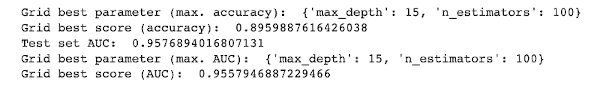From this output, we conclude that for good accuracy, the best parameters are ‘max_depth’ equals 15 and ‘n_estimators’ equals 100, which gives the accuracy score of 89.59%. If you use ‘roc_auc’ scoring, the best parameters are ‘max_depth’ equals 15 and ‘n_estimators’ equals 100 resulting in the accuracy score of 95.57%. On test data, we get an accuracy of 95.76%.

“Gradient boosting is a machine learning technique for regression and classification problems, which produces a prediction model in the form of an ensemble of weak prediction models, typically decision trees.” - Wikipedia, Gradient boosting

The Gradient Boost algorithm is applied using following code snippet:

``````# classifier

# parameters
param = {'learning_rate' : [.05,.1]}

# model
grid_clf_acc2 = GridSearchCV(clf2, param_grid = param)

# train the model
grid_clf_acc2.fit(X_train, y_train)

print('Grid best parameter (max. accuracy): ', grid_clf_acc2.best_params_)
print('Grid best score (accuracy): ', grid_clf_acc2.best_score_)

# alternative metric to optimize over grid parameters: AUC
grid_clf_auc2 = GridSearchCV(clf2, param_grid = param, scoring = 'roc_auc')
grid_clf_auc2.fit(X_train, y_train)
predict_proba = grid_clf_auc2.predict_proba(X_test)[:,1]

print('Test set AUC: ', roc_auc_score(y_test, predict_proba))
print('Grid best parameter (max. AUC): ', grid_clf_auc2.best_params_)
print('Grid best score (AUC): ', grid_clf_auc2.best_score_)
``````

This is the output :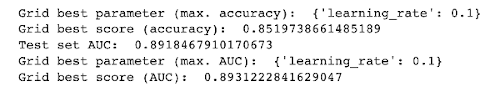From the above output, we observe that the best ‘learning_rate’ is 0.1. The accuracy score is 85.19%. For ‘roc_auc’ score, the best ‘learning_rate’ is once again 0.1. The accuracy score is 89.31%. The accuracy score on the test dataset is 89.18%.

## MLP Classifier (A Neural Network Classifier)

“A multilayer perceptron (MLP) is a class of feedforward artificial neural network (ANN). The term MLP is used ambiguously, sometimes loosely to any feedforward ANN, sometimes strictly to refer to networks composed of multiple layers of perceptrons (with threshold activation). Multilayer perceptrons are sometimes colloquially referred to as "vanilla" neural networks, especially when they have a single hidden layer.” - Wikipedia - Multilayer perceptron

The MLPClassfier is applied using following code:

``````# classifier
clf3 = MLPClassifier(max_iter=1000)

# scaling training and test sets
from sklearn.preprocessing import MinMaxScaler
scaler = MinMaxScaler()
X_train_scaled = scaler.fit_transform(X_train)
X_test_scaled = scaler.transform(X_test)

# parameters
param = {'hidden_layer_sizes' : [10,100,[10,10]], 'activation' : ['tanh','relu'], 'solver' : ['adam','lbfgs']}

# model
grid_clf_acc3 = GridSearchCV(clf3, param_grid = param)

# train the model
grid_clf_acc3.fit(X_train_scaled, y_train)

print('Grid best parameter (max. accuracy): ', grid_clf_acc3.best_params_)
print('Grid best score (accuracy): ', grid_clf_acc3.best_score_)

# alternative metric to optimize over grid parameters: AUC
grid_clf_auc3 = GridSearchCV(clf3, param_grid = param, scoring = 'roc_auc')
grid_clf_auc3.fit(X_train_scaled, y_train)
predict_proba = grid_clf_auc3.predict_proba(X_test_scaled)[:,1]

print('Test set AUC: ', roc_auc_score(y_test, predict_proba))
print('Grid best parameter (max. AUC): ', grid_clf_auc3.best_params_)
print('Grid best score (AUC): ', grid_clf_auc3.best_score_)
``````

This is the output :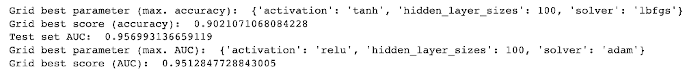From the above output, we observe that the best parameter values are ‘activation’ equals tanh, ‘hidden_layer_sizes’ equals 100 and ‘solver’ is lbfgs. The accuracy score is 90.21%. For ‘roc_auc’ score, the best parameters values are - ‘activation’ equals tanh, ‘hidden_layer_sizes’ equals 100, and ‘solver’ equals lbfgs. The accuracy score is 95.12%. The accuracy score on the test dataset is 95.69%.

## Comparing Results

I have tabulated the results of the above training, which is shown here.

Random Forest Gradient Boost MLP Classifier
Best Score (accuracy) 0.8959 0.8519 0.9021
Best Score AUC 0.9557 0.8931 0.9512
Test Set AUC 0.9576 0.8918 0.9569

Obviously, MLP classifiers outperform the other two. So, we will use this one for inference.

## Inference

We do the predictions on our test dataset by calling the `predict` method:

``````pred = grid_clf_auc3.predict(X_test_scaled)
``````

We examine the first five predictions.

``````pred[:5]
``````

This is the output :

``````array([0, 0, 0, 0, 1])
``````

The value 0 in the above output shows that there will not be a connection between the nodes at the corresponding index in the future. Similarly, the value of 1 would show a probable future connection.

Do not worry, if this looks confusing, I will explain this further shortly. Before that let us look at the accuracy score on our predictions.

## Accuracy Score

Print the accuracy score

``````accuracy_score(pred,y_test)
``````

This is the output :

``````0.9049050632911393
``````

We observe that our predictions are 90.49% accurate. Let us try the confusion matrix for evaluation.

## Confusion Matrix

Generate the confusion matrix:

``````confusion_matrix(pred,y_test)
``````

This is the output :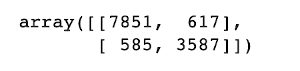From the confusion matrix we can see that 11438 values (7851 (True Positive) + 3587 (True Negative)) are correct and 1202 values (617 (False Positive) + 585 (False Negative)) are incorrect.

We do further analysis using roc_auc and plotting the ROC curve.

## The ROC_AUC Score and ROC Curve

ROC - AUC curve is a performance measurement for classification problems at various thresholds settings. ROC gives the probability and AUC represents degree or measure of separability. This shows the model’s capability to distinguish between classes. Higher the AUC, better the model is at predicting 0s as 0s and 1s as 1s.

We consider the areas under the ROC curve - excellent for AUC values between 0.9-1, good for AUC values between 0.8-0.9, fair for AUC values between 0.7-0.8, poor for AUC values between 0.6-0.7 and bad for AUC values between 0.5-0.6.

Compute roc_auc score:

``````predict_proba = grid_clf_auc3.predict_proba(X_test_scaled)[:,1]

false_positive_rate,true_positive_rate,_ = roc_curve(y_test, predict_proba)
roc_auc_score = auc(false_positive_rate,true_positive_rate)
``````

Plot ROC curve:

``````plt.plot(false_positive_rate,true_positive_rate)
plt.title(f'ROC Curve \n ROC AUC Score : {roc_auc_score}')
plt.xlabel('False Positive Rate')
plt.ylabel('True Positive Rate')
``````

This is the output :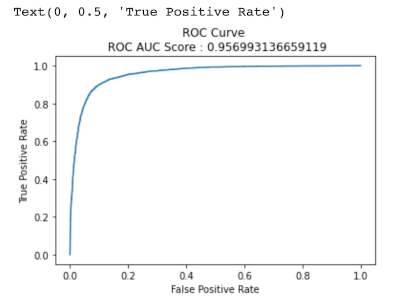From the above plot, the area under the ROC curve (AUC) produces a value greater than 0.95, this shows that our classifier is close to being a perfect classifier, i.e. the predictions are accurate.

## Demonstration

Now, we come to a real use-case of our entire analysis so far. We will find the probability of two nodes forming an edge.

First let’s look at our dataframe.

``````df1.head()
``````

This is the output :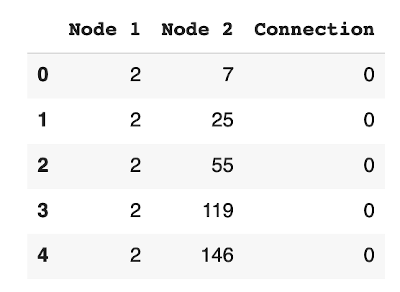Let’s choose nodes 2 and 146. These nodes do not have an edge between them denoted by ‘0’ in the connection column.

We need to get the features of the node pair (2,146) from the ‘X’ array, containing all the node pair features, which we had created earlier, and we need to find the index of this node pair features in the `X_train` array.

Note : Features for node pairs (2, 146) are in `X_train` but some node pair features will be in `X_test`, since X has been divided into `X_train` and `X_test`.

``````print(f' ({df1.iloc[4,0]},{df1.iloc[4,1]}) node pair features : {X}')

# its position in X_train
print(f'Index of ({df1.iloc[4,0]},{df1.iloc[4,1]}) node pair in X_train : {np.where(X_train == X)}')
``````

This is the output :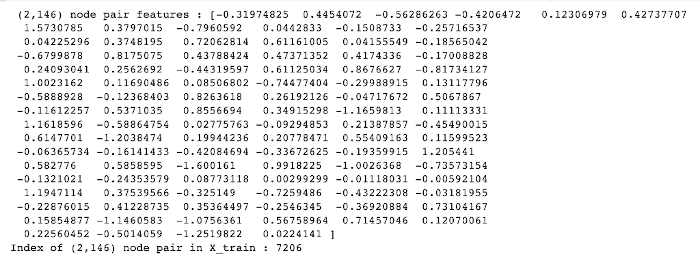You can see that the index for node pair (2,146) in `X_train` is 7206. Now we are going to predict the probability of an edge forming between these two nodes in the future, using the `predict_proba` function. We will be using `X_train_scaled` since we have used the scaled version to train our model. `X_train` and `X_train_scaled` have values at the same indices.

``````predict_proba = grid_clf_auc3.predict_proba(X_train_scaled[np.where(X_train == X)].reshape(1,-1))[:,1]

print(f'Probability of nodes {df1.iloc[4,0]} and {df1.iloc[4,1]} to form a link is : {float(predict_proba)*100 : .2f}%')
``````

This is the output :

``````Probability of nodes 2 and 146 to form a link is :  9.09%
``````

From the output we can see that the probability of an edge to form between nodes 2 and 146 is 9.09%.

One has to choose a threshold value for giving suggestions. Let’s say we choose 80%, in that case, we will tell the user that the likelihood of a future connection between these nodes is very high.

Thus, we can conclude that using node2vec and a machine learning model, we can do the prediction on a future connection between any two nodes in a humongous network.

## Saving Dataset

The `df1` data frame contains the list of the existing and non-existing edges, which will be required for the next part, part 3 of this series.

``````df1.to_csv('/content/Facebook-Social-Network-Analysis-Project-master/df1.csv, index=False')
``````

# Summary

In this second part of the tutorial, you started with the network graph created in the first part. You generated the adjacency matrix on this graph to find the node pairs which do not have an edge. We chose 4000 node pairs randomly, which do not have an edge, and kept only this node pairs having a path between them. Then you saw how to create a graph which we assume to be existing at a previous point of time and assume our original graph as the graph which will be generated in the future. We did this so we can use the new graph for training and the original graph for predicting.

In this part you also learnt how to use node2vec for getting the features of our nodes. We added the features of the nodes of the particular node pair to get the features of the edges/node pairs. Next, you saw how one can use GridSearchCV to find out the best classifier for our model among RandomForest, Gradient Boost and MLP classifiers, using unique sets of parameters for a classifier. You also learnt how to plot the ROC Curve of a model which helps us to know how well our model is performing on the test data.

That’s all. Try out the node2vec module in your social network analysis projects and see how well it is working on your graph. Happy Coding!